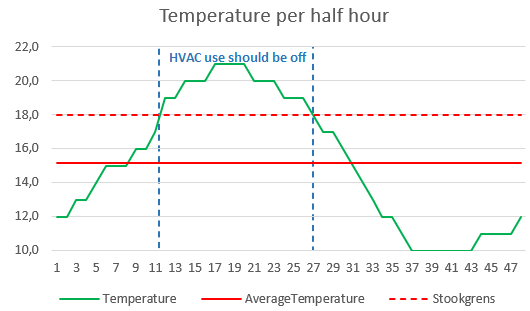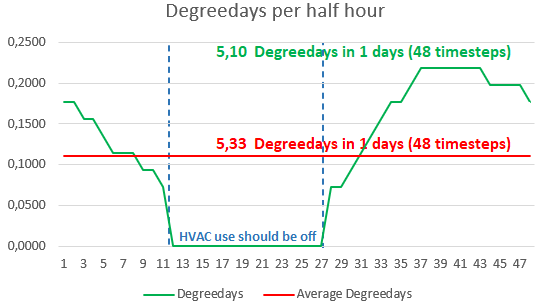Degreedays were introduced by a Dutch oil company early in the sixties of the former century with the purpose to calculate the expected use of fuel in buildings of their clients. Later on degreedays (and cooling degreedays) were used to analyse the energyperformance of buildings, to predict plant and animal development rates (growing degreedays) or to predict when mosquitoes and black fly emerge and cease.

In this article we will show that with our meteodata it is possible to calculate the degreedays in a more refined way when using the smaller timesteps between our meteodata. An innovative Energy Saving COmpany was in 2003 the first and is still the only one to vary the indoor temperature with respect the building and HVAC use. In this way they could measure energy saving performance more accurately.

Calculating classical unweighted degreedaysHere the focus however lies upon the use of degreedays for energy management purposes.

The Dutch Wikipedia page of Degreedays uses the following (translated from Dutch) definition:

A degree day is defined as reference temperature (18 ° C) minus the average temperature over the day, minimized at 0. The average temperature over a day in the Netherlands is typically measured at the KNMI in De Bilt. If the average temperature over a given day was 10 degrees Celsius, then that day has an equivalent of 8 degrees days. If the average temperature is higher than the reference temperature (for example, 20 degrees), then typically no heating is required; the number of degree days is then 0 (and not -2). Typically degree days are summed over an entire year. In the Netherlands there are about 3000 degree days per year.

The classical degreedays can thus be calculated with the following formula:

1. Degreedays = Indoor Temperature - Outdoor Temperature  if  Outdoor Temperature < Indoor Temperature - Heating Limit
2. Degreedays = 0  if Outdoor Temperature >= Indoor Temperature - Heating Limit

With the Indoor Temperature is set to 20,5 °C, the Heating Limit is set to 2,5 °C and the Outdoor Temperature the Degreedays can be simply calculated.

Evaluating classical degreedaysThree problems arise when calculating degreedays:

1. The Indoor Temperature differs for the various buildings. For instance a swimmingpool (28 °C) has a higher Average Indoor Temperature than an office (20,5 °C). The innovative Energy Saving COmpany (ESCO) Connerga was in 2003 the first and is still the only one to vary the indoor temperature with respect the building and HVAC use. In this way they could measure energy saving performance more accurately.
2. The Outdoor Temperature changes in time. Therefore using a Dayly Average Outdoor Temperature will lead to inconsistencies. In this article the effects of calculating degreedays with smaller timesteps than a day will be discussed.
3. The energy content of gas varies through the year and with respect to the outdoor temperature.
4. The influence of other meteorological parameters than temperature.

Weighted degreedays

You can correct for the effects of the energy content of gas or the effect of other meteorological parameters than temperature by multiplying degreedays with the following weightfactor:

1. April till septembre: 0.8
2. March and octobre: 1.0
3. Novembre till February: 1.1

The use of weighted degreedays should be considered carefully with respect of the physical circumstances.

Calculating degreedays with smaller timesteps than a day

Degreedays with the timestep T2 - T1 can be calculated with the following formula:

1. Degreedays = (Indoor Temperature - Outdoor Temperature) * (T2 - T1)  if  Outdoor Temperature < Indoor Temperature - Heating Limit in timestep T2 - T1
2. Degreedays = 0  if Outdoor Temperature >= Indoor Temperature - Heating Limit in timestep T2 - T1

In the figures below the difference between calculating degreedays with a daily average temperature and a temperature measured each half hour is illustrated (for a specific weatherstation on a specific day). When the temperature measured each half hour rises above Indoor Temperature - Heating Limit the Degreedays will be 0. The difference in degreedays when using daily average temperture and the temperature measured each half hour is in this specific case 4.3%. When calculating the energy savings this difference can be of great importance in order to calculate the savings as exactly as possible.